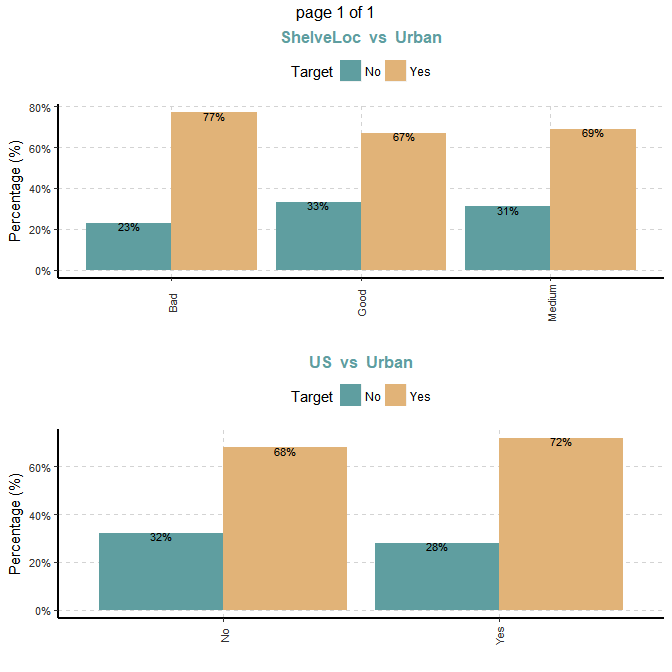# Summarize and Explore the Data

## Intro

The document introduces the SmartEDA package and how it can help you to build exploratory data analysis.

SmartEDA includes multiple custom functions to perform initial exploratory analysis on any input data describing the structure and the relationships present in the data. The generated output can be obtained in both summary and graphical form. The graphical form or charts can also be exported as reports.

सर्वस्य लोचनं शास्त्रं
Science is the only eye

अनेकसंशयोच्छेदि, परोक्षार्थस्य दर्शक|
सर्वस्य लोचनं शास्त्रं, यस्य नास्त्यन्ध एव सः ||

It blasts many doubts, foresees what is not obvious |
Science is the eye of everyone, one who hasn't got it, is like a blind ||

SmartEDA package helps you to construct a good base of data understanding. The capabilities and functionalities are listed below

1. SmartEDA package will make you capable of applying different types of EDA without having to
• remember the different R package names
• write lengthy R scripts
• manual effort to prepare the EDA report
2. No need to categorize the variables into Character, Numeric, Factor etc. SmartEDA functions automatically categorize all the features into the right data type (Character, Numeric, Factor etc.) based on the input data.

3. ggplot2 functions are used for graphical presentation of data

4. Rmarkdown and knitr functions were used for build HTML reports

To summarize, SmartEDA package helps in getting the complete exploratory data analysis just by running the function instead of writing lengthy r code.

## Data

In this vignette, we will be using a simulated data set containing sales of child car seats at 400 different stores.

Data Source ISLR package.

Install the package “ISLR” to get the example data set.

install.packages("ISLR")
library("ISLR")
install.packages("SmartEDA")
library("SmartEDA")
## Load sample dataset from ISLR pacakge
Carseats= ISLR::Carseats

### Overview of the data

Understanding the dimensions of the dataset, variable names, overall missing summary and data types of each variables

# Overview of the data - Type = 1
ExpData(data=Carseats,type=1,DV=NULL)

# Structure of the data - Type = 2
ExpData(data=Carseats,type=2,DV=NULL)
• Overview of the data
Descriptions Obs
Total Sample 400
No. of Variables 11
No. of Numeric Variables 8
No. of Factor Variables 3
No. of Text Variables 0
No. of Date Variables 0
No. of Zero variance Variables (Uniform) 0
%. of Variables having complete cases 100%
%. of Variables having <50% missing cases 0%
%. of Variables having >50% missing cases 0%
%. of Variables having >90% missing cases 0%
• Structure of the data
S.no VarName VarClass VarType
1 Sales numeric Independet variable
2 CompPrice numeric Independet variable
3 Income numeric Independet variable
5 Population numeric Independet variable
6 Price numeric Independet variable
7 ShelveLoc* factor Independet variable
8 Age numeric Independet variable
9 Education numeric Independet variable
10 Urban* factor Independet variable
11 US* factor Independet variable

## Exploratory data analysis (EDA)

This function shows the EDA output for 3 different cases

1. Target variable is not defined
2. Target variable is continuous
3. Target variable is categorical

### Example for case 1: Target variable is not defined

#### 1.1 Summary of numerical variables

Summary of all numerical variables

ExpNumStat(Carseats,by="A",gp=NULL,Qnt=seq(0,1,0.1),MesofShape=2,Outlier=TRUE,round=2)

#### 1.2 Distributions of numerical variables

Graphical representation of all numeric features

• Density plot (Univariate)
# Note: Variable excluded (if unique value of variable which is less than or eaual to 10 [nlim=10])
ExpNumViz(Carseats,gp=NULL,nlim=10,Page=c(2,2),sample=8)
## $0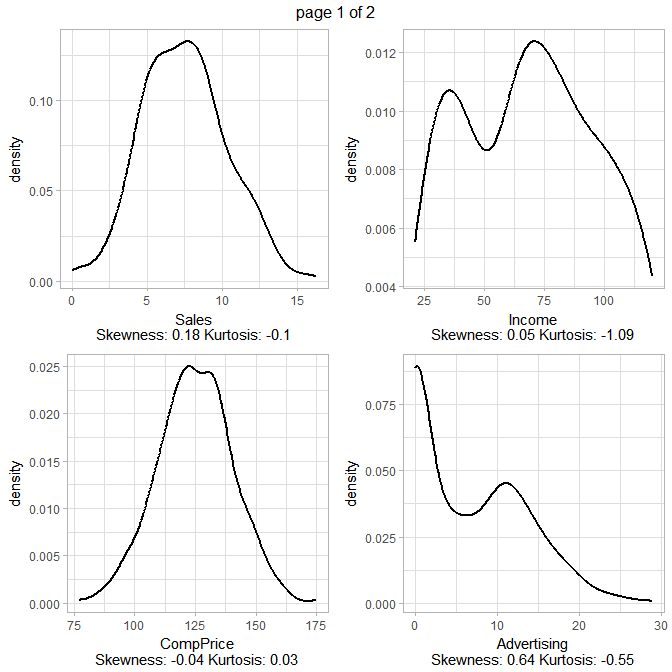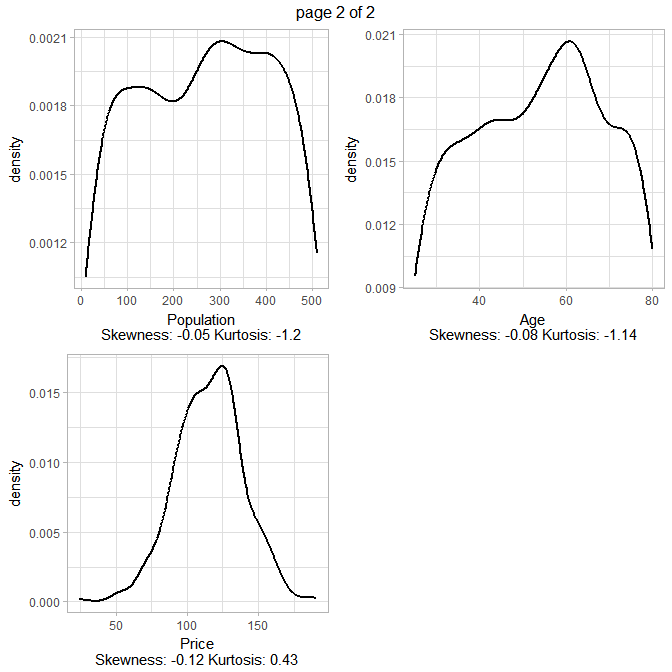#### 1.3. Summary of categorical variables • frequency for all categorical independent variables ExpCTable(Carseats,Target=NULL,margin=1,clim=10,nlim=NULL,round=2,bin=NULL,per=T) Variable Valid Frequency Percent CumPercent ShelveLoc Bad 96 24.00 24.00 ShelveLoc Good 85 21.25 45.25 ShelveLoc Medium 219 54.75 100.00 ShelveLoc TOTAL 400 NA NA Urban No 118 29.50 29.50 Urban Yes 282 70.50 100.00 Urban TOTAL 400 NA NA US No 142 35.50 35.50 US Yes 258 64.50 100.00 US TOTAL 400 NA NA NA is Not Applicable #### 1.4. Distributions of categorical variables • Bar plots for all categorical variables ExpCatViz(Carseats,gp=NULL,fname=NULL,clim=10,margin=2,Page = c(2,1),sample=4) ##$0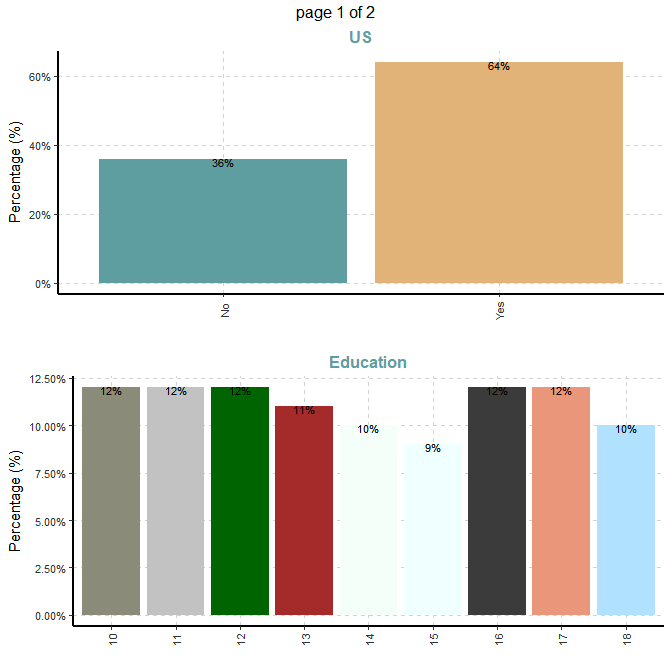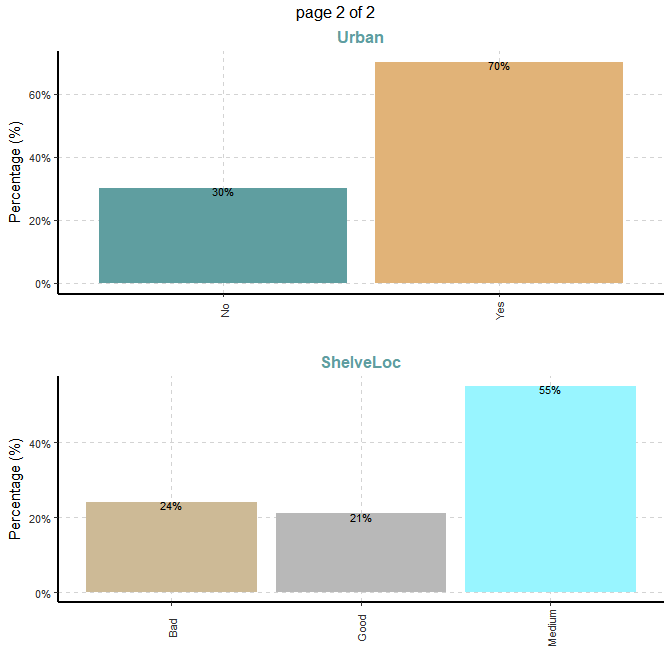### Example for case 2: Target variable is continuous

#### 2.1. Target variable

Summary of continuous dependent variable

1. Variable name - Price
2. Variable description - Price company charges for car seats at each site
summary(Carseats[,"Price"])
##    Min. 1st Qu.  Median    Mean 3rd Qu.    Max.
##    24.0   100.0   117.0   115.8   131.0   191.0

#### 2.2 Summary of numerical variables

Summary statistics when dependent variable is continuous Price.

ExpNumStat(Carseats,by="A",gp="Price",Qnt=seq(0,1,0.1),MesofShape=1,Outlier=TRUE,round=2)

If Target variable is continuous, summary statistics will add the correlation column (Correlation between Target variable vs all independet variables)

#### 2.3 Distributions of numerical variables

Graphical representation of all numeric variables

• Scatter plot (Bivariate)

Scatter plot between all numeric variables and target variable Price. This plot help to examine how well a target variable is correlated with dependent variables.

Dependent variable is Price (continuous).

#Note: sample=8 means randomly selected 8 scatter plots
#Note: nlim=4 means included numeric variable with unique value is more than 4
ExpNumViz(Carseats,gp="Price",nlim=4,fname=NULL,col=NULL,Page=c(2,2),sample=8)
## $0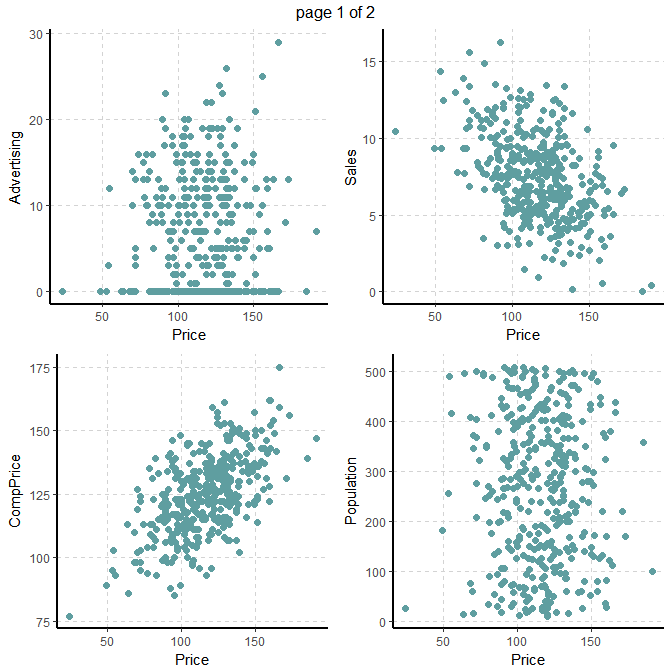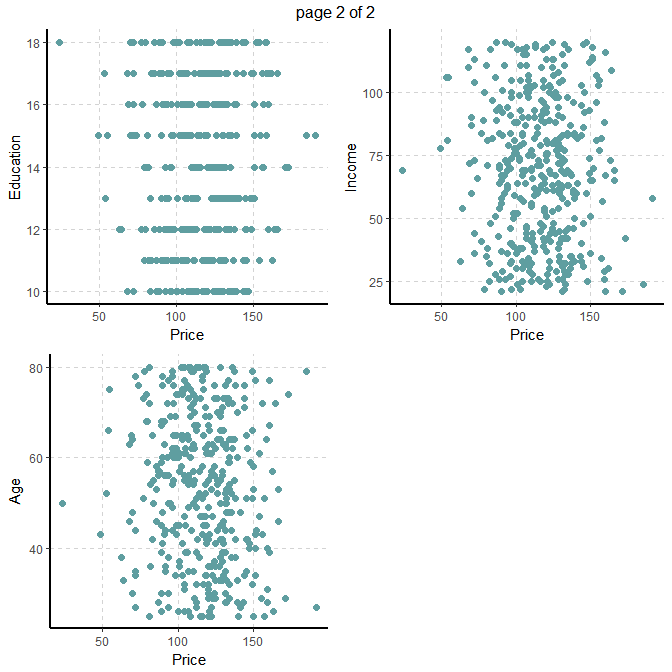#### 2.4. Summary of categorical variables Summary of categorical variables • frequency for all categorical independent variables by descretized Price ##bin=4, descretized 4 categories based on quantiles ExpCTable(Carseats,Target="Price",margin=1,clim=10,nlim=NULL,round=2,bin=4,per=F) ### Example for case 3: Target variable is categorical #### 3.1. Target variable Summary of categorical dependent variable 1. Variable name - Urban 2. Variable description - Whether the store is in an urban or rural location Urban Frequency Descriptions No 118 Store location Yes 282 Store location #### 3.1 Summary of numerical variables Summary of all numeric variables ExpNumStat(Carseats,by="GA",gp="Urban",Qnt=seq(0,1,0.1),MesofShape=2,Outlier=TRUE,round=2) #### 3.2 Distributions of Numerical variables • Box plots for all numerical variables vs categorical dependent variable - Bivariate comparision only with categories Boxplot for all the numeric attributes by each category of Urban ExpNumViz(Carseats,gp="Urban",type=1,nlim=NULL,fname=NULL,col=c("pink","yellow","orange"),Page=c(2,2),sample=8) ##$0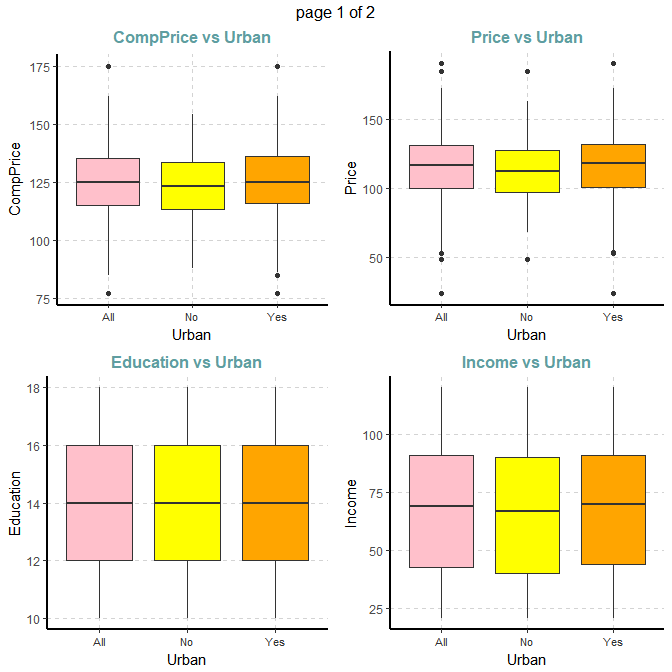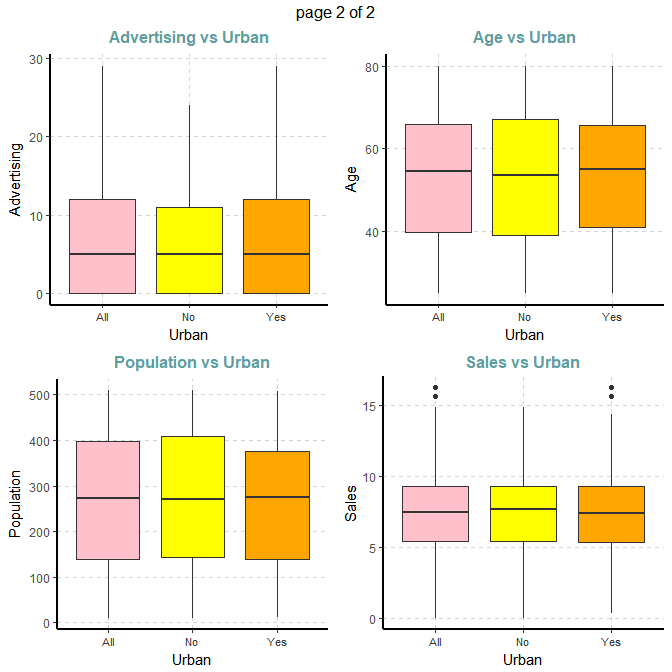#### 3.3 Summary of categorical variables

Cross tabulation with target variable

• Custom tables between all categorical independent variables and target variable Urban
ExpCTable(Carseats,Target="Urban",margin=1,clim=10,nlim=NULL,round=2,bin=NULL,per=F)
VARIABLE CATEGORY Urban:No Urban:Yes TOTAL
ShelveLoc Good 28 57 85
ShelveLoc Medium 68 151 219
ShelveLoc TOTAL 118 282 400
US No 46 96 142
US Yes 72 186 258
US TOTAL 118 282 400

Information Value

ExpCatStat(Carseats,Target="Urban",Label="Store Location",result = "IV",clim=10,nlim=5,Pclass="Yes")
Variable Target Class Out_1 Out_0 TOTAL Per_1 Per_0 Odds WOE IV Ref_1 Ref_0
ShelveLoc Urban Bad 74 22 96 0.262 0.186 1.409 0.343 0.026 Yes No
ShelveLoc Urban Good 57 28 85 0.202 0.237 0.852 -0.160 0.006 Yes No
ShelveLoc Urban Medium 151 68 219 0.535 0.576 0.929 -0.074 0.003 Yes No
US Urban No 96 46 142 0.340 0.390 0.872 -0.137 0.007 Yes No
US Urban Yes 186 72 258 0.660 0.610 1.082 0.079 0.004 Yes No

Statistical test

ExpCatStat(Carseats,Target="Urban",Label="Store Location",result = "Stat",clim=10,nlim=5,Pclass="Yes")
Variable Target Unique Chi-squared p-value df IV Value Pred Power
ShelveLoc Urban 3 2.738 0.254 2 0.035 Somewhat Predictive
US Urban 2 0.684 0.408 1 0.011 Not Predictive

#### 3.4. Distributions of categorical variables

Stacked bar plot with vertical or horizontal bars for all categorical variables

ExpCatViz(Carseats,gp="Urban",fname=NULL,clim=10,col=NULL,margin=2,Page = c(2,1),sample=2)
## \$0